ENGR338 Lab 2022 Fall
Lab 5
The Inverter

The Inverter

Introduction: This lab uses Electric VLSI and LTSpice to simulate pmos and nmos gates in order to create an inverter.

Materials: LTSpice, Electric VLSI

Methods: First, an NMOS with size 10/2 and a PMOS with size 20/2 were placed in series. A voltage source and ground were placed accordingly and off-page connectors were placed between the transistors to complete the circuit. An icon view was created and a DRC check was performed as seen in Figure 1.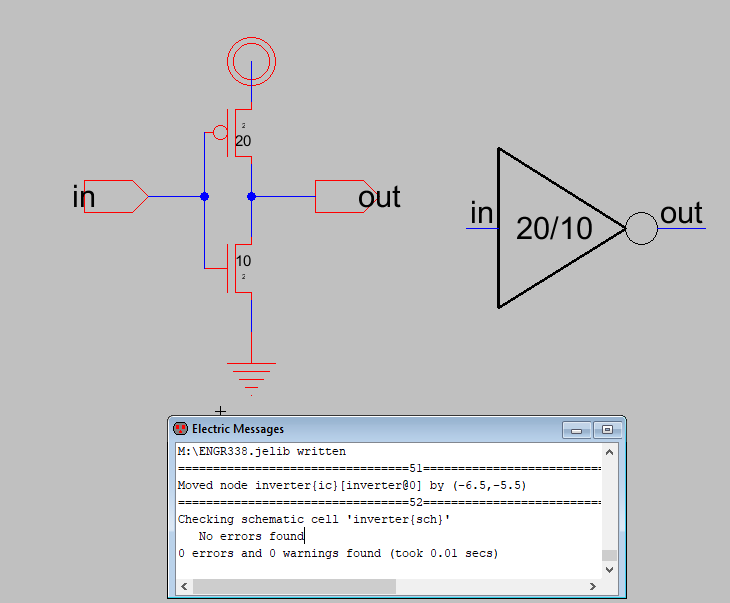Figure 1. Inverter Schematic and Icon with 0 DRC Errors.

The circuit was then simulated in LTSpice as seen in Figure 2.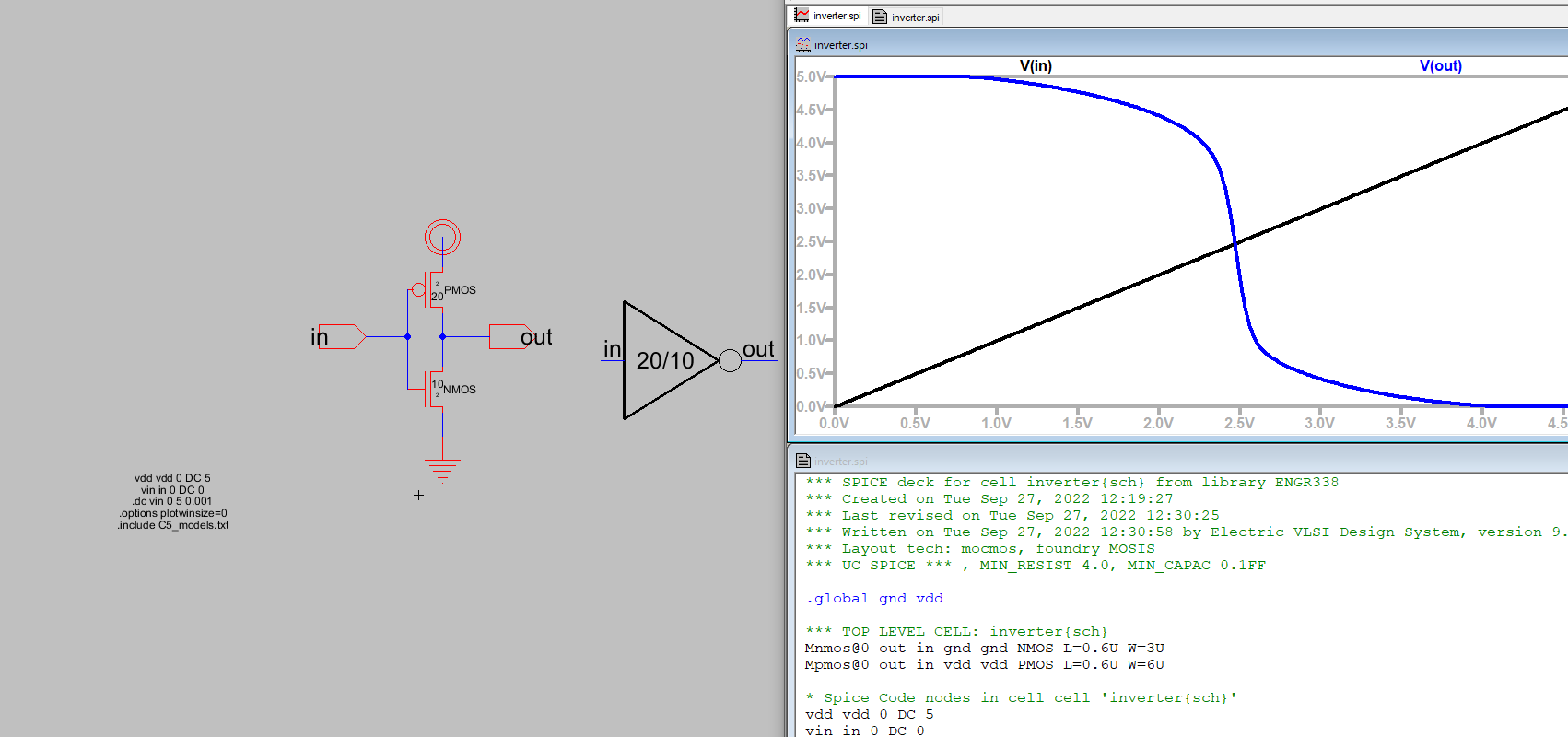Figure 2. Inverter Simulation.

A transient analysis using a pulse function was then run which can be seen below in Figure 3.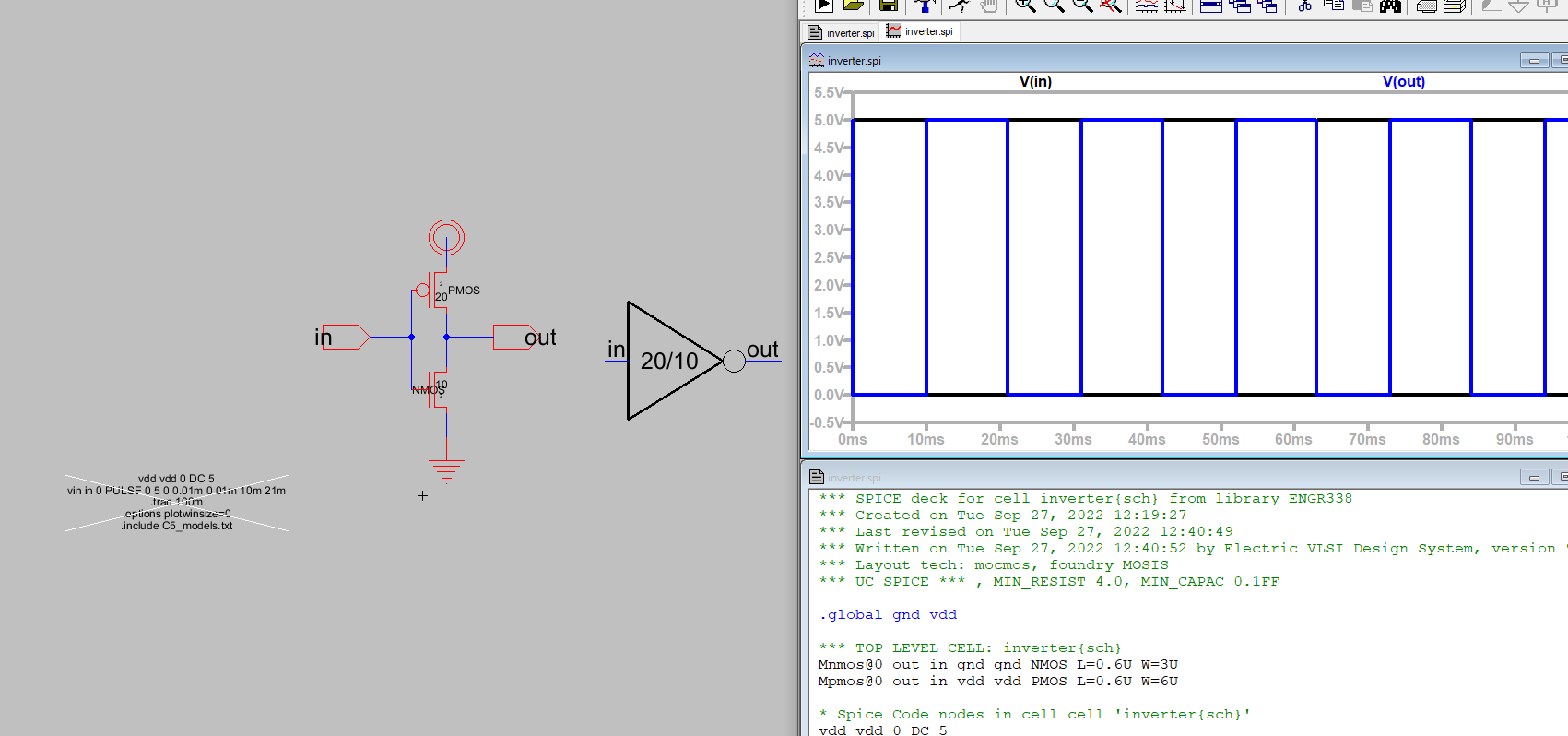Figure 3. Inverter Pulse Simulation.

The layout was created for the schematic which went fairly smoothly with few issues and can be seen below in Figure 4.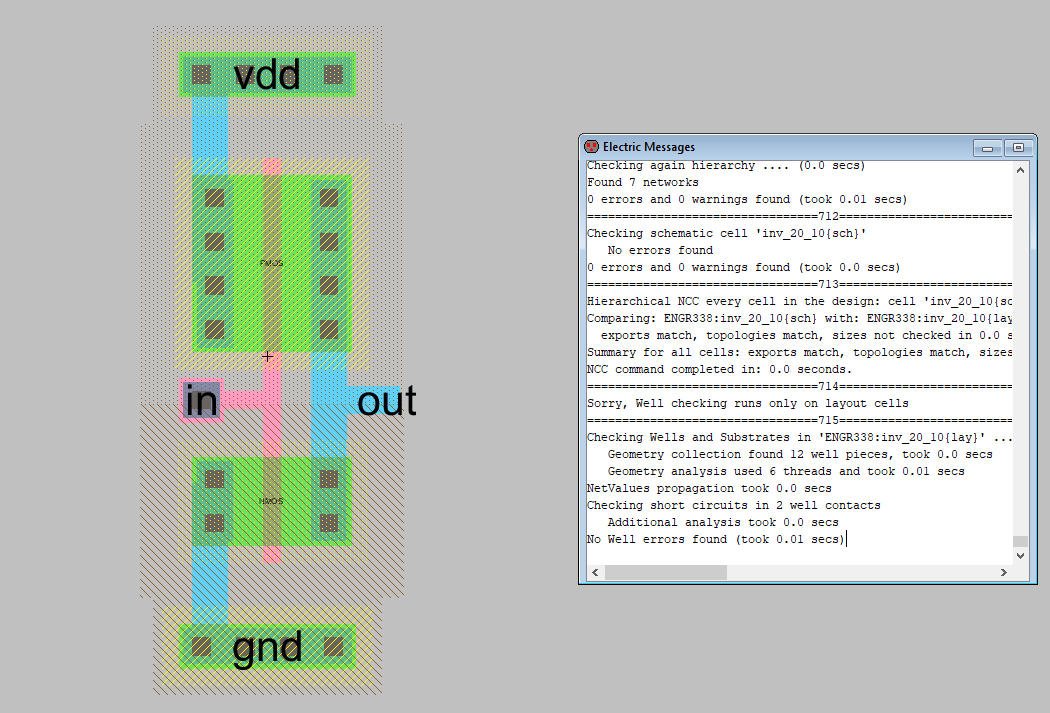Figure 3. Inverter Schematic with Well Check, DRC, and NCC complete.

The inverter was then multiplied by creating a duplicate schematic and using the Spice multiplier function to create an inverter with M=5 forming a 100/2 and 50/2 inverter by putting 5 of our previous inverters in parallel(I forgot to grab a screengrab of the schematic). The layout was then completed by copying the previous schematic 4 times. The VDD and GND rails width was increased to encompas the circuit and all of the transistors were wired as seen below in Figure 4.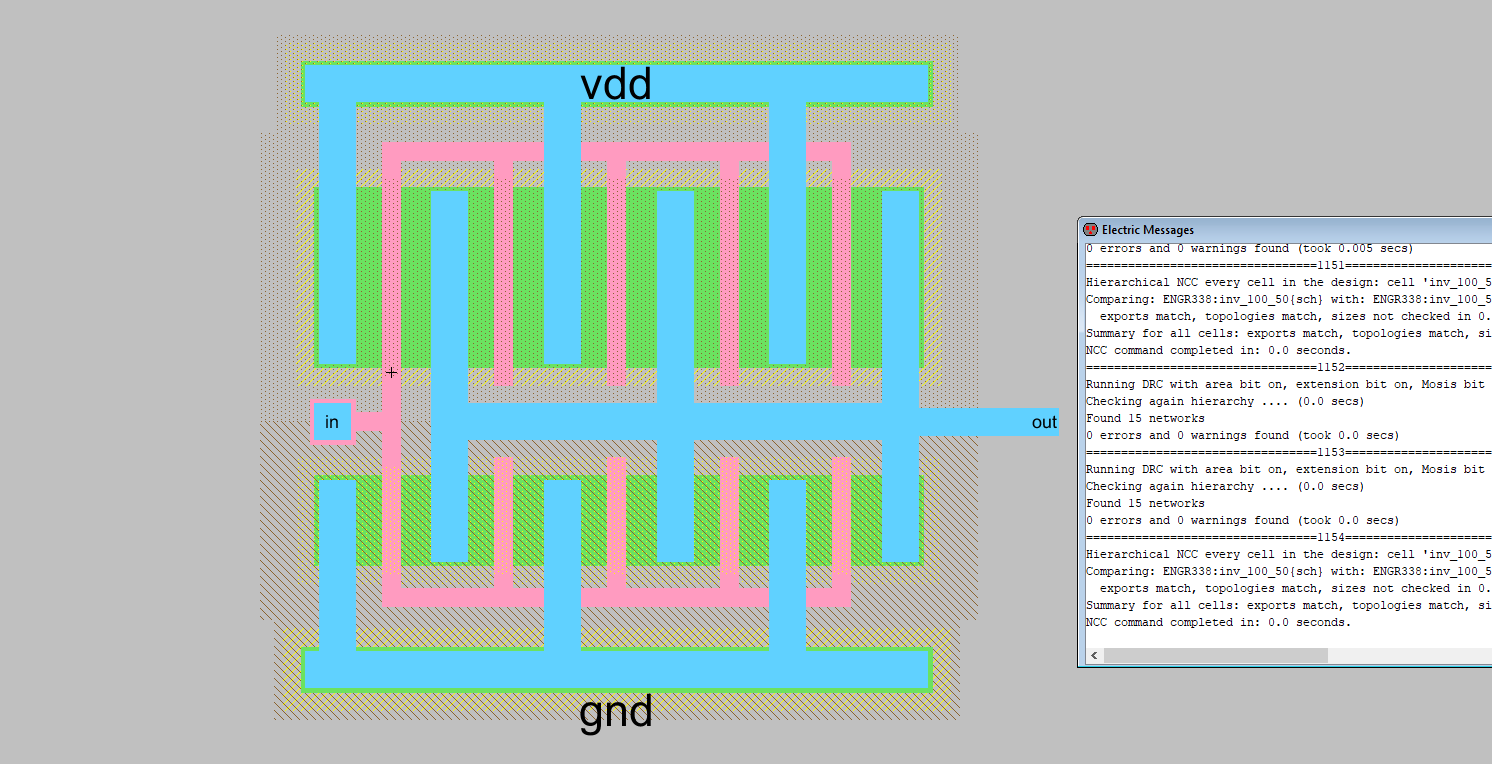Figure 4. Inverter Schematic with Well Check, DRC, and NCC complete.

The circuits were then wired to drive various capacitors to compare the maximum current through the two gates.

Results:

Both circuits were wired to drive a 100fF capacitor, the results can be seen below in Figure 5 and 6.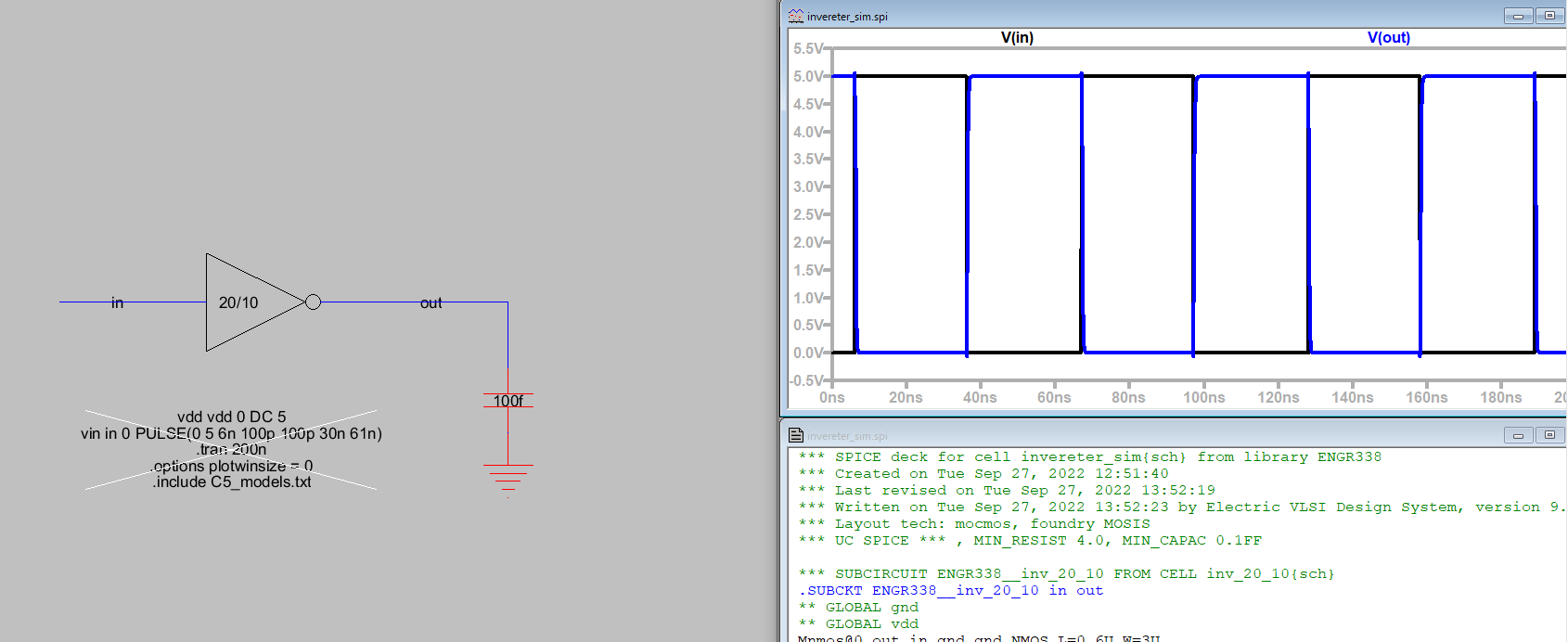Figure 5. Simulation
results for the 20/10 inverter gate wired to a 100fF capacitor.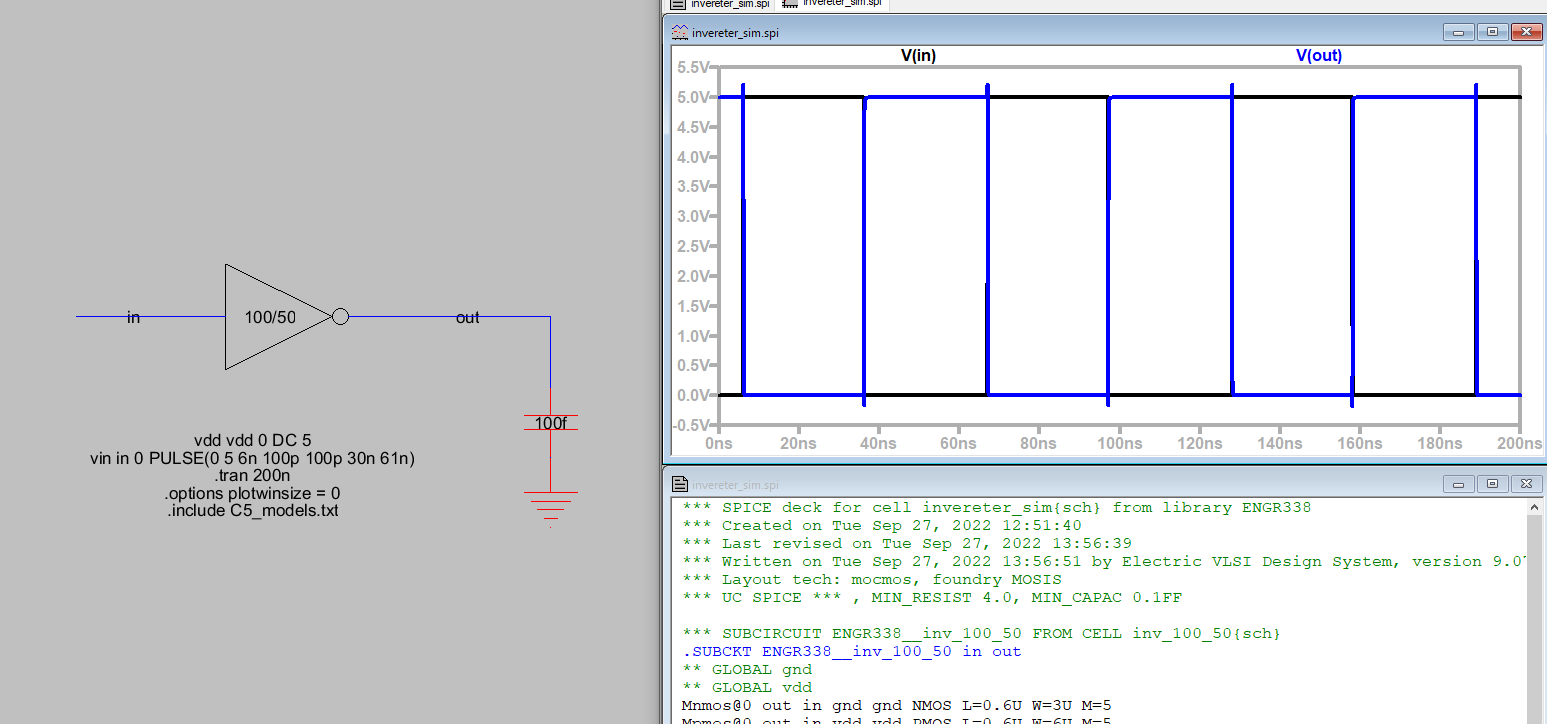Figure 6. Simulation results for the 100/50 inverter gate wired to a 100fF capacitor.

Both circuits were then wired to drive a 1pF capacitor, the results can be seen below in Figure 5 and 6.Figure 7. Simulation
results for the 20/10 inverter gate wired to a 1pF capacitor.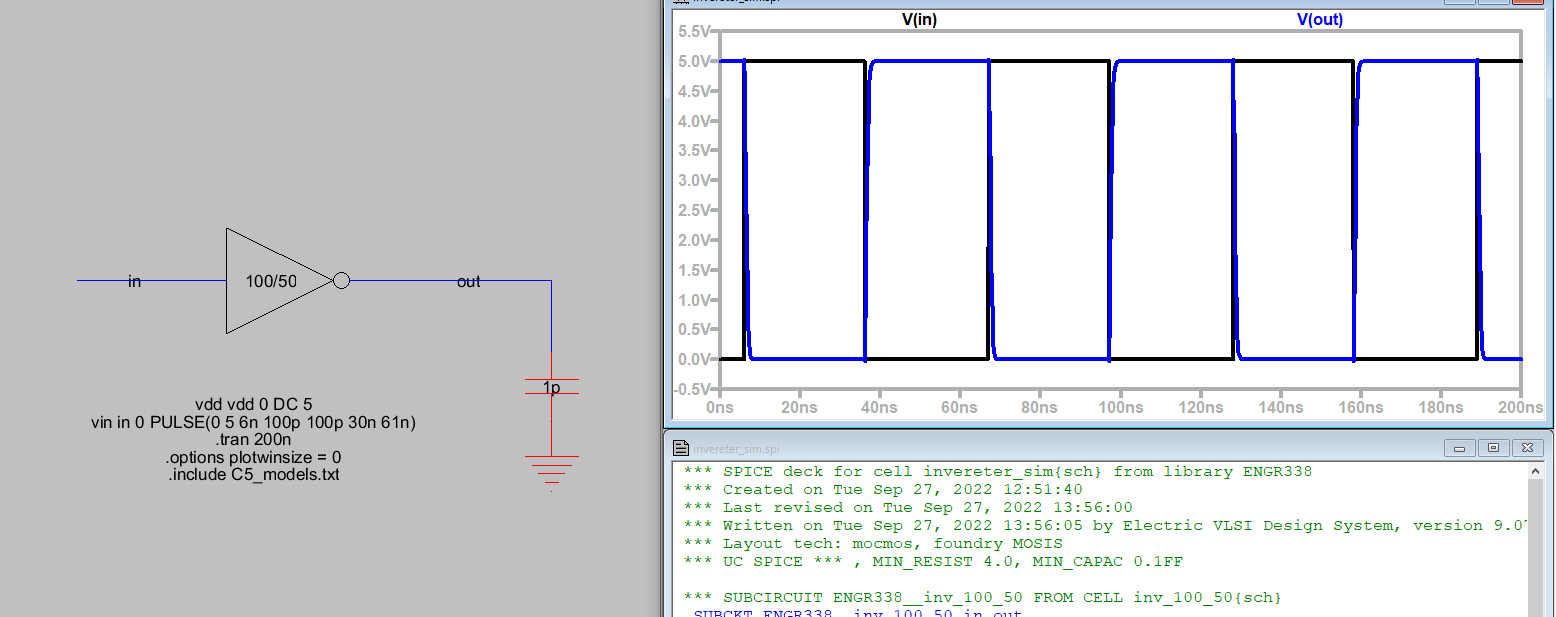Figure 8. Simulation results for the 100/50 inverter gate wired to a 1pF capacitor.

Finally, both circuits were wired to drive a 10pF capacitor, the results can be seen below in Figure 9 and 10.Figure 9. Simulation
results for the 20/10 inverter gate wired to a 100fF capacitor.Figure 10. Simulation results for the 100/50 inverter gate wired to a 10pF capacitor.

Discussion: This lab was fairly straightforward once the correct spacing was achieved for the 100/20 inverter. I found the easiest approach to getting the spacing right was to use the info panel and adjust the x position through that. Otherwise, this lab was fairly straight forward and the results self explanatory.